﻿

### A note on some homology spheres which are 2-fold coverings of inequivalent knots

#### Abstract

We construct a family of closed 3--manifolds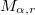$M_{\alpha,r}$, which are  homeomorphic to the Brieskorn homology spheres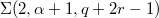$\Sigma(2, \alpha+1, q+2r-1)$,  where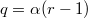$q=\alpha(r-1)$ and both$\alpha \ge 1$ and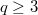$q \ge 3$ are odd. We show  that$M_{\alpha,r}$ can be represented as 2--fold covering of the 3--sphere  branched over two inequivalent knots. Our proofs follow immediately from two  different symmetries of a genus 2 Heegaard diagram of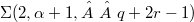$\Sigma(2, \alpha+1, q+2r-1)$, and generalize analogous results proved in [BGM], [IK], [SIK] and  [T].

DOI Code: 10.1285/i15900932v30n1p41

Keywords:
3–manifold; branched covering; orbifold; fundamental group; homology 3–sphere; (1, 1)-knot; torus knot

Classification: 57M05; 57M12; 57R65

Full Text: PDFThis work is licensed under a Creative Commons Attribuzione - Non commerciale - Non opere derivate 3.0 Italia License.